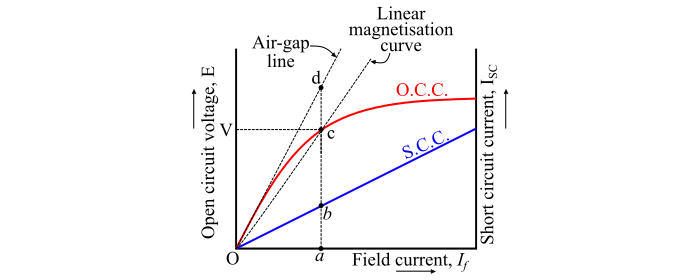# Saturated and Unsaturated Synchronous Reactance in Synchronous Generator

## Unsaturated Synchronous Reactance

At low excitations, the open-circuit characteristic (OCC) of an alternator coincides with the air-gap line, thus, the synchronous impedance of the alternator is constant and this value of the synchronous impedance is known as linear or unsaturated synchronous impedance.

The unsaturated synchronous reactance (denoted by Xsu) can be obtained from the air-gap line voltage and the short-circuit current of the alternator for a particular value of the field current.NoteSCC is the short-circuit characteristic.

From the characteristics, the unsaturated synchronous impedance is given by,

$$\mathrm{𝑍_{𝑠𝑢} =\frac{𝐸_{𝑎𝑑}}{𝐼_{𝑎𝑏}}= 𝑅_{𝑎} + 𝑗𝑋_{𝑠𝑢}}$$

If the armature resistance of the alternator is neglected, then the unsaturated synchronous reactance is given by,

$$\mathrm{X_{𝑠𝑢} =\frac{𝐸_{𝑎𝑑}}{𝐼_{𝑎𝑏}}}$$

## Saturated Synchronous Reactance

With the increasing excitation, the effect of the saturation decreases the value of synchronous impedance and hence the values of the synchronous impedance beyond the linear part of the OCC are known as saturated synchronous impedances. The values of the saturated synchronous impedance are not constant but vary with the excitation and the power factor of the alternator on load.

The approximate value of the synchronous reactance varies with the degree of saturation of the open-circuit characteristic. Hence, the value of the synchronous reactance to be used in a given problem should be one calculated at the approximate load on the alternator. If the alternator is connected to the infinite bus, then its terminal voltage remains the same at the bus value.

If the field current of the machine is changed, the excitation voltage will change – not along the open-circuit characteristic, but along the line Oc (refer the above figure) that is known as modified air-gap line. This modified air-gap line represents the same magnetic saturation level as that corresponding to the operating point ‘c’. Therefore, the saturated synchronous reactance at the rated voltage is obtained as follows −

$$\mathrm{𝑍_{𝑠\:(sat.)} =\frac{𝐸_{𝑎𝑐}}{𝐼_{𝑎𝑏}}= 𝑅_{𝑎 }+ 𝑗𝑋_{𝑠(sat.)}}$$

If the armature resistance is neglected, then the saturated synchronous reactance is given by,

$$\mathrm{𝑋_{𝑠\:(sat.)} =\frac{𝐸_{𝑎𝑐}}{𝐼_{𝑎𝑏}}}$$